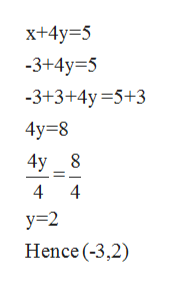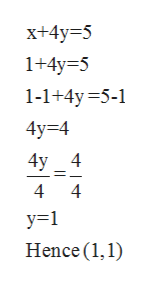Consider the following equation. x+4y=5Use the given x-coordinates {-3,1 ,5 } to find solution points that should be plotted when graphing this equation. (Select all that apply.)(1,1)(5,0)(-3,2)(1,5)(5,-3)(-3,4)

Question

Consider the following equation.

x+4y=5

Use the given x-coordinates {-3,1 ,5 } to find solution points that should be plotted when graphing this equation. (Select all that apply.)

(1,1)

(5,0)

(-3,2)

(1,5)

(5,-3)

(-3,4)

Step 1

x+4y=5

Use the given x-coordinates {-3,1 ,5 } to find solution points that should be plotted when graphing this equation.

Step 2

At x=-3help_outlineImage Transcriptionclosex+4y 5 -3+4y 5 -3+3+4y=5+3 4y 8 4y 8 4 4 y 2 Hence (-3,2) fullscreen
Step 3

At x=1

...help_outlineImage Transcriptionclosex+4y 5 1+4y 5 1-1+4y 5-1 4у%34 4y 4 4 4 у-1 Hence (1,1) fullscreen

Want to see the full answer?

See Solution

Want to see this answer and more?

Our solutions are written by experts, many with advanced degrees, and available 24/7

See Solution
Tagged in

Linear Programming